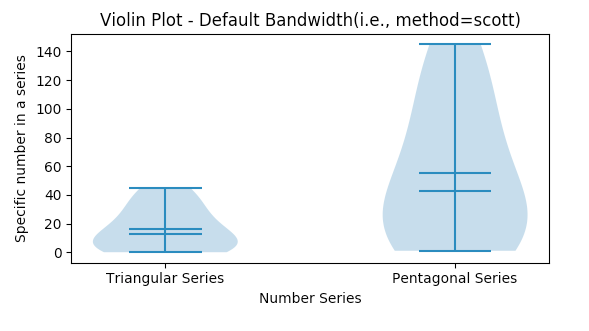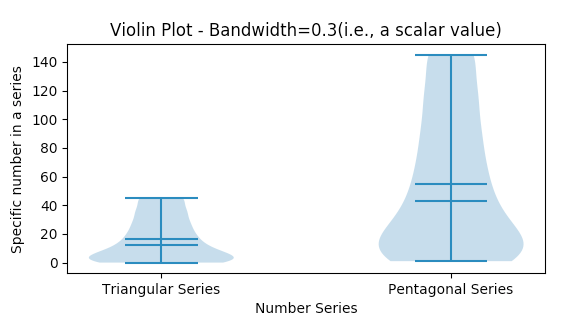# Drawing A Violin Plot Using Python And Matplotlib

## Overview:

• A violin plot combines two aspects of a distribution in a single visualization:
• The features of a Box Plot: Median, Interquartile Distance
• The Probability Density Function
• In a violin plot, the Probability Density Function-PDF of the distribution is tilted side wards and placed on both the sides of the box plot.
• A violin plot clearly displays the multiple modes present in a multi-modal data.
• Violin plots display the whole distribution. They do not display outliers separately as in case of Box plots.

## Drawing a violin plot using Python and Matplotlib:

• To create a violin plot, import the matplotlib.pyplot module and call the method violinplot() function by passing the data as sequences.
• The violin plot can be customized to display mean and median values.
• Using Matplotlib both vertical and horizontal violin plots can be created through the parameter vert. By default the value of the vert parameter is True, which creates a vertical violin plot. The width of the violin can be controlled by the widths parameter.

## Example:

 # Example python program to draw a violin plot using matplotlib import matplotlib.pyplot as plot import pandas as pd import numpy as np   # Customized method to draw violin plot in a matplotlib subplot def drawViolinPlot(axis, xlabel, xticks, xticklabels, ylabel, bandwidth, title):     # Customize x-axis     axis.set_xlabel(xlabel);     axis.set_xticks(xticks);     axis.set_xticklabels(xticklabels);       # Customize y-axis     axis.set_ylabel(ylabel);       # Draw a violin plot for the distributions - Default bandwidth     axis.violinplot(sequences, showmeans=True, showmedians=True, bw_method=bandwidth);     axis.set_title(title);     # Distributions as python lists triangularSeries = [0, 1, 3, 6, 10, 15, 21, 28, 36, 45]; pentagonalSeries = [1, 5, 12, 22, 35, 51, 70, 92, 117, 145];   # A tuple of distributions sequences = (triangularSeries, pentagonalSeries);   figure, axis = plot.subplots(2, 1); plot.subplots_adjust(hspace=1);   bandwidth = None; drawViolinPlot(axis,               "Number Series",               np.arange(len(sequences)+1),               ('', 'Triangular Series', 'Pentagonal Series'),               "Specific number in a series", bandwidth,               "Violin Plot - Default Bandwidth(i.e., method=scott)");   bandwidth = 0.3; drawViolinPlot(axis,               "Number Series",               np.arange(len(sequences)+1),               ('', 'Triangular Series', 'Pentagonal Series'),               "Specific number in a series", bandwidth,               "Violin Plot - Bandwidth=0.3(i.e., a scalar value)");                # Display violin plot plot.show();

## Output: